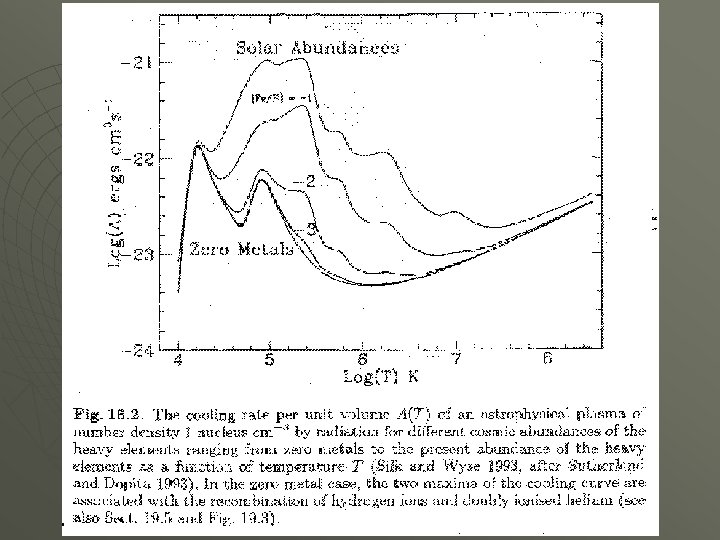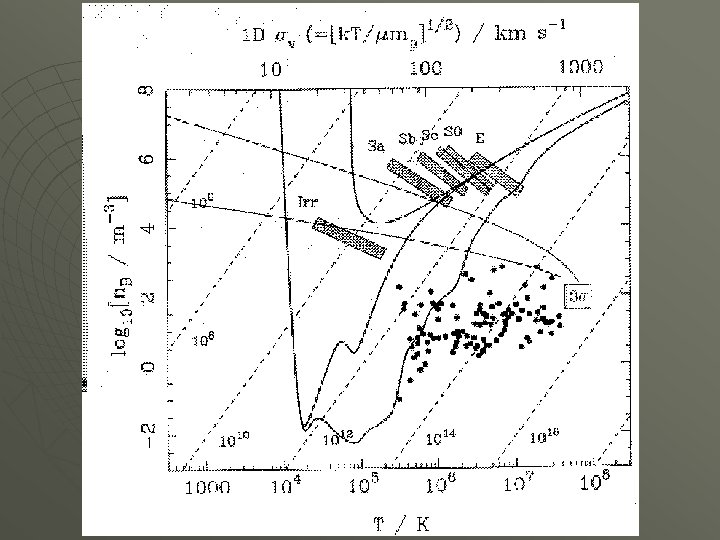Скачать презентацию The Galaxy Formation Paradigm Remember to mention pdf

a4ce2450d566b29d2fc8f11f2618c32a.ppt

• Количество слайдов: 27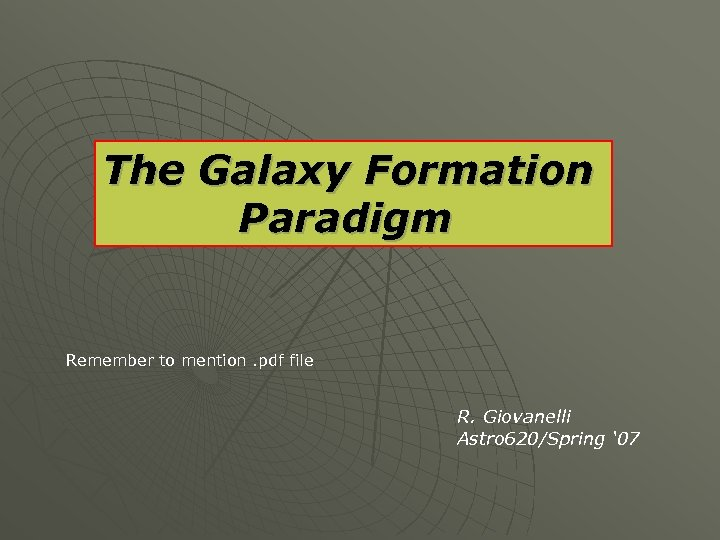The Galaxy Formation Paradigm Remember to mention. pdf file R. Giovanelli Astro 620/Spring ‘ 07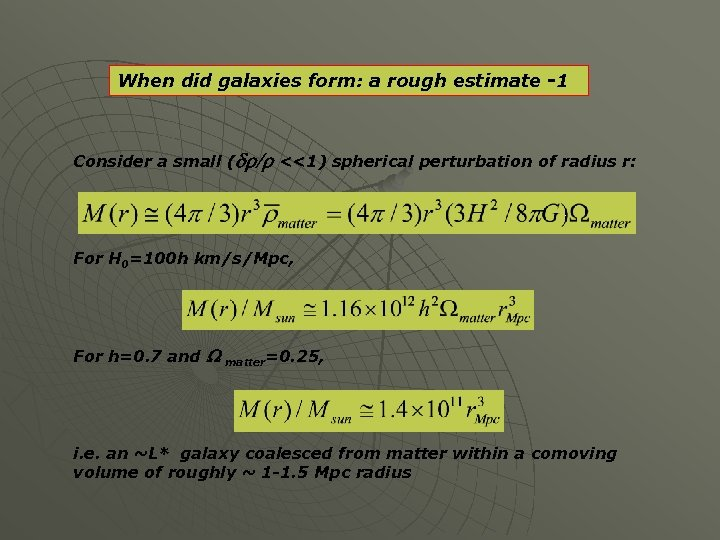When did galaxies form: a rough estimate -1 Consider a small (dr/r <<1) spherical perturbation of radius r: For H 0=100 h km/s/Mpc, For h=0. 7 and W matter=0. 25, i. e. an ~L* galaxy coalesced from matter within a comoving volume of roughly ~ 1 -1. 5 Mpc radius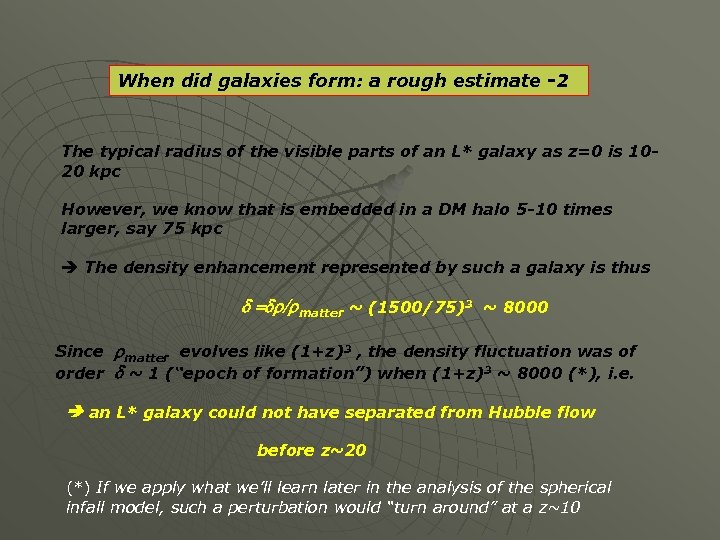When did galaxies form: a rough estimate -2 The typical radius of the visible parts of an L* galaxy as z=0 is 1020 kpc However, we know that is embedded in a DM halo 5 -10 times larger, say 75 kpc The density enhancement represented by such a galaxy is thus d =dr/rmatter ~ (1500/75)3 ~ 8000 Since rmatter evolves like (1+z)3 , the density fluctuation was of order d ~ 1 (“epoch of formation”) when (1+z)3 ~ 8000 (*), i. e. an L* galaxy could not have separated from Hubble flow before z~20 (*) If we apply what we’ll learn later in the analysis of the spherical infall model, such a perturbation would “turn around” at a z~10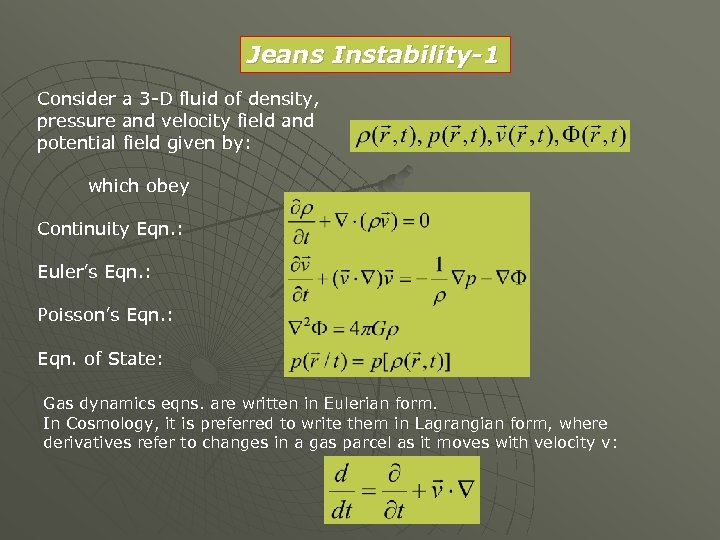Jeans Instability-1 Consider a 3 -D fluid of density, pressure and velocity field and potential field given by: which obey Continuity Eqn. : Euler’s Eqn. : Poisson’s Eqn. : Eqn. of State: Gas dynamics eqns. are written in Eulerian form. In Cosmology, it is preferred to write them in Lagrangian form, where derivatives refer to changes in a gas parcel as it moves with velocity v: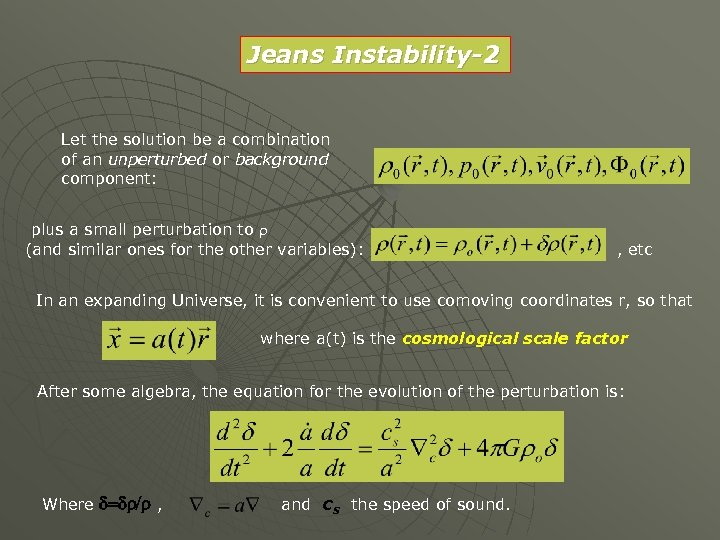Jeans Instability-2 Let the solution be a combination of an unperturbed or background component: plus a small perturbation to r (and similar ones for the other variables): , etc In an expanding Universe, it is convenient to use comoving coordinates r, so that where a(t) is the cosmological scale factor After some algebra, the equation for the evolution of the perturbation is: Where d=dr/r , and cs the speed of sound.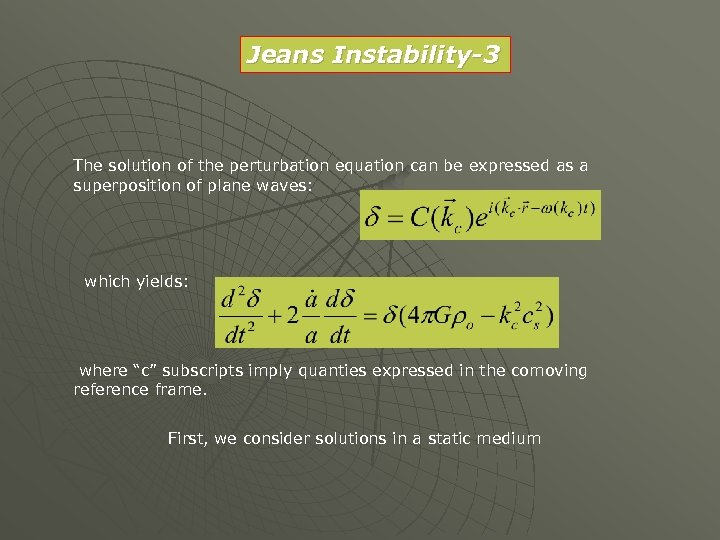Jeans Instability-3 The solution of the perturbation equation can be expressed as a superposition of plane waves: which yields: where “c” subscripts imply quanties expressed in the comoving reference frame. First, we consider solutions in a static medium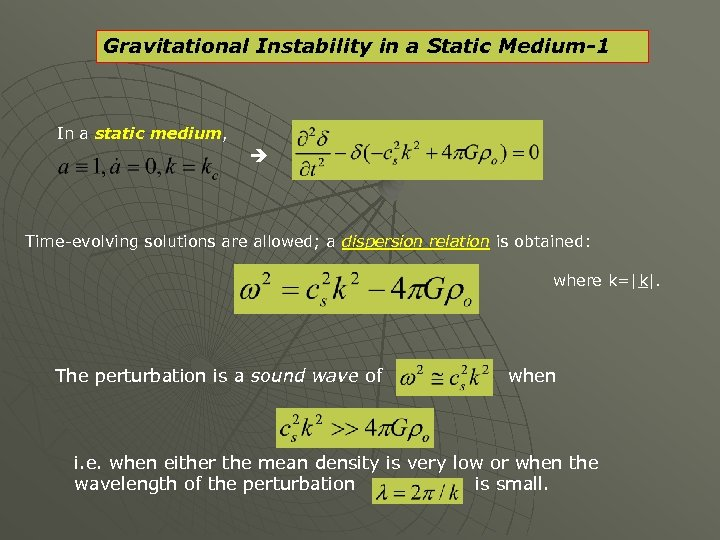Gravitational Instability in a Static Medium-1 In a static medium, Time-evolving solutions are allowed; a dispersion relation is obtained: where k=|k|. The perturbation is a sound wave of when i. e. when either the mean density is very low or when the wavelength of the perturbation is small.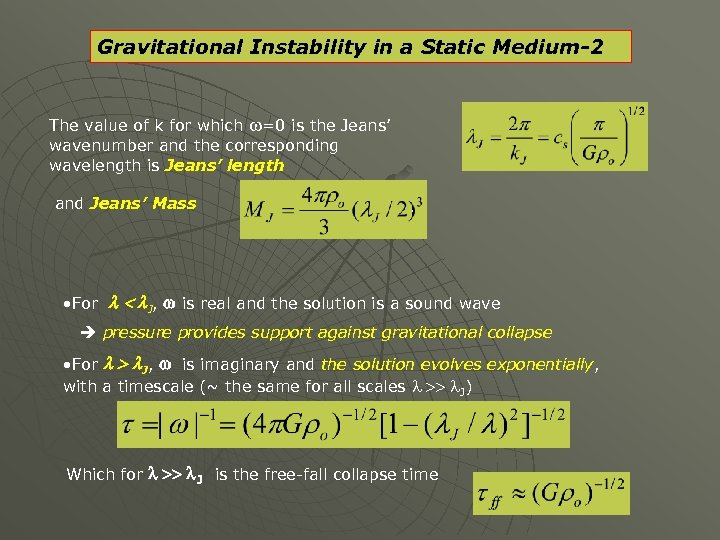Gravitational Instability in a Static Medium-2 The value of k for which w=0 is the Jeans’ wavenumber and the corresponding wavelength is Jeans’ length and Jeans’ Mass • For l < l. J, w is real and the solution is a sound wave pressure provides support against gravitational collapse • For l > l. J, w is imaginary and the solution evolves exponentially, with a timescale (~ the same for all scales l >> l. J) Which for l >> l. J is the free-fall collapse time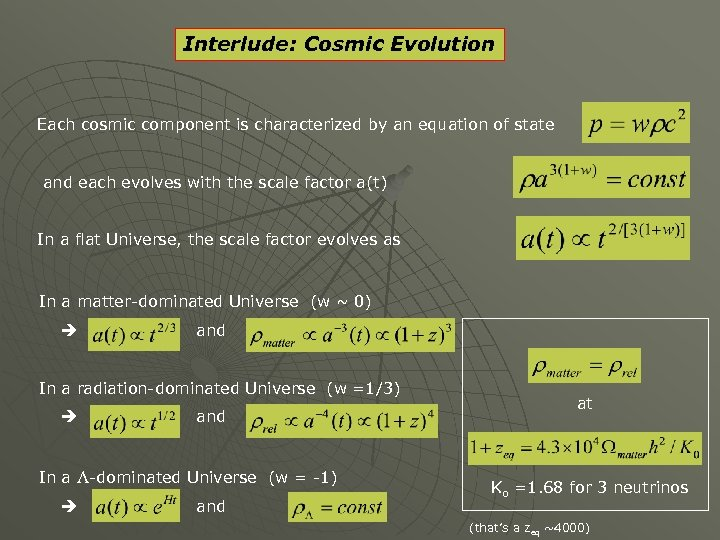Interlude: Cosmic Evolution Each cosmic component is characterized by an equation of state and each evolves with the scale factor a(t) In a flat Universe, the scale factor evolves as In a matter-dominated Universe (w ~ 0) and In a radiation-dominated Universe (w =1/3) and In a L-dominated Universe (w = -1) and at Ko =1. 68 for 3 neutrinos (that’s a zeq ~4000)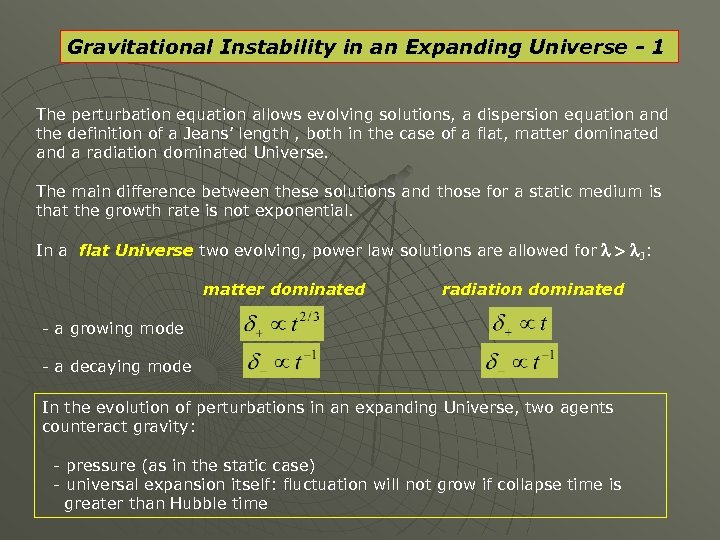Gravitational Instability in an Expanding Universe - 1 The perturbation equation allows evolving solutions, a dispersion equation and the definition of a Jeans’ length , both in the case of a flat, matter dominated and a radiation dominated Universe. The main difference between these solutions and those for a static medium is that the growth rate is not exponential. In a flat Universe two evolving, power law solutions are allowed for l > l. J: matter dominated radiation dominated - a growing mode - a decaying mode In the evolution of perturbations in an expanding Universe, two agents counteract gravity: - pressure (as in the static case) - universal expansion itself: fluctuation will not grow if collapse time is greater than Hubble time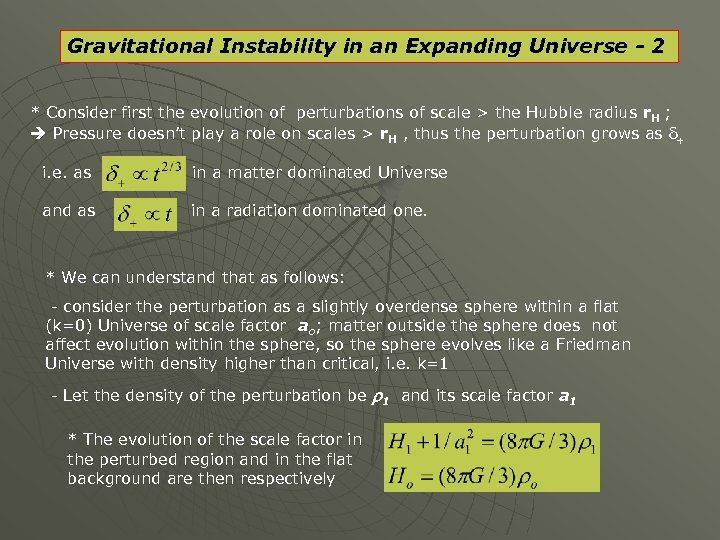Gravitational Instability in an Expanding Universe - 2 * Consider first the evolution of perturbations of scale > the Hubble radius r. H ; Pressure doesn’t play a role on scales > r. H , thus the perturbation grows as d+ i. e. as in a matter dominated Universe and as in a radiation dominated one. * We can understand that as follows: - consider the perturbation as a slightly overdense sphere within a flat (k=0) Universe of scale factor ao; matter outside the sphere does not affect evolution within the sphere, so the sphere evolves like a Friedman Universe with density higher than critical, i. e. k=1 - Let the density of the perturbation be r 1 and its scale factor a 1 * The evolution of the scale factor in the perturbed region and in the flat background are then respectively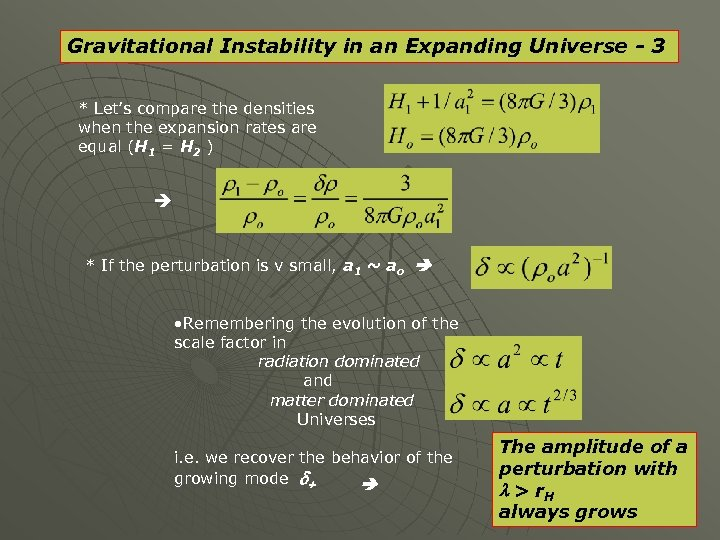Gravitational Instability in an Expanding Universe - 3 * Let’s compare the densities when the expansion rates are equal (H 1 = H 2 ) * If the perturbation is v small, a 1 ~ ao • Remembering the evolution of the scale factor in radiation dominated and matter dominated Universes i. e. we recover the behavior of the growing mode d+ The amplitude of a perturbation with l > r. H always grows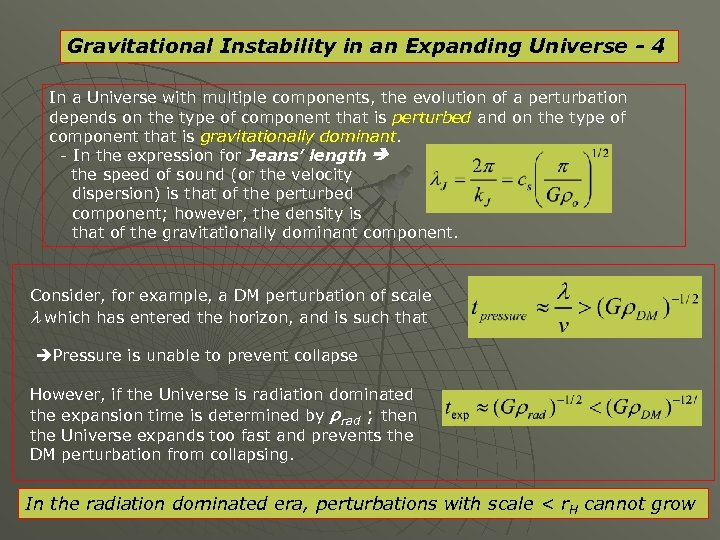Gravitational Instability in an Expanding Universe - 4 In a Universe with multiple components, the evolution of a perturbation depends on the type of component that is perturbed and on the type of component that is gravitationally dominant. - In the expression for Jeans’ length the speed of sound (or the velocity dispersion) is that of the perturbed component; however, the density is that of the gravitationally dominant component. Consider, for example, a DM perturbation of scale l which has entered the horizon, and is such that Pressure is unable to prevent collapse However, if the Universe is radiation dominated the expansion time is determined by rrad ; then the Universe expands too fast and prevents the DM perturbation from collapsing. In the radiation dominated era, perturbations with scale < r. H cannot grow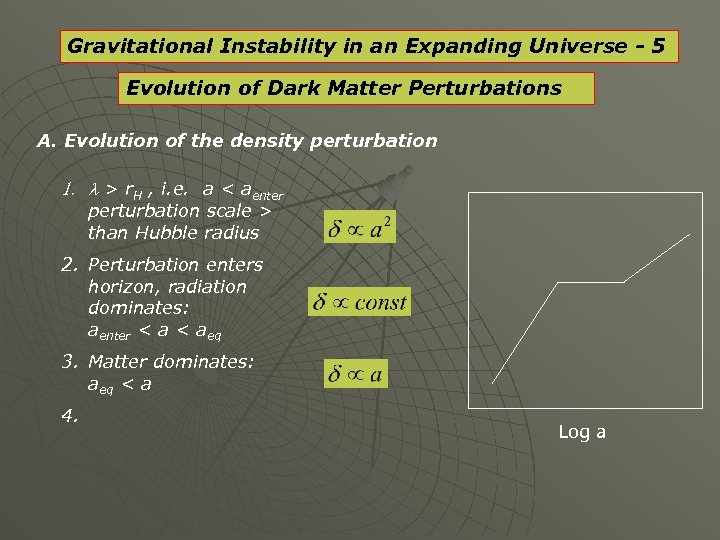Gravitational Instability in an Expanding Universe - 5 Evolution of Dark Matter Perturbations A. Evolution of the density perturbation 1. l > r. H , i. e. a < aenter perturbation scale > than Hubble radius 2. Perturbation enters horizon, radiation dominates: aenter < aeq 3. Matter dominates: aeq < a 4. Log a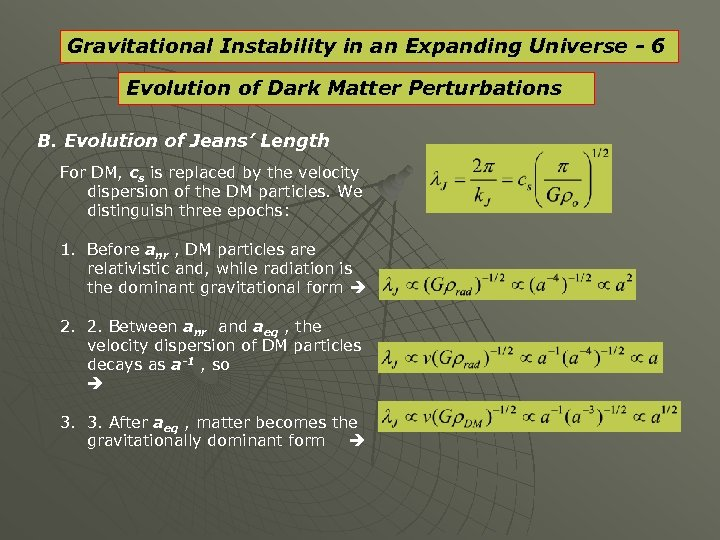Gravitational Instability in an Expanding Universe - 6 Evolution of Dark Matter Perturbations B. Evolution of Jeans’ Length For DM, cs is replaced by the velocity dispersion of the DM particles. We distinguish three epochs: 1. Before anr , DM particles are relativistic and, while radiation is the dominant gravitational form 2. 2. Between anr and aeq , the velocity dispersion of DM particles decays as a-1 , so 3. 3. After aeq , matter becomes the gravitationally dominant form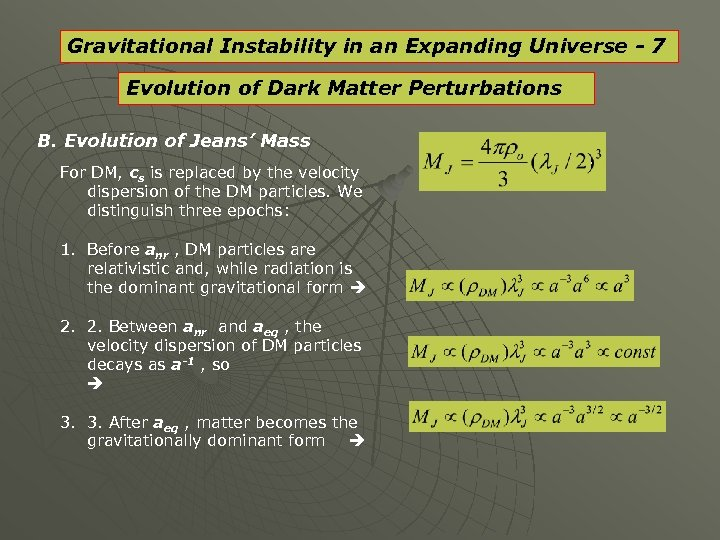Gravitational Instability in an Expanding Universe - 7 Evolution of Dark Matter Perturbations B. Evolution of Jeans’ Mass For DM, cs is replaced by the velocity dispersion of the DM particles. We distinguish three epochs: 1. Before anr , DM particles are relativistic and, while radiation is the dominant gravitational form 2. 2. Between anr and aeq , the velocity dispersion of DM particles decays as a-1 , so 3. 3. After aeq , matter becomes the gravitationally dominant form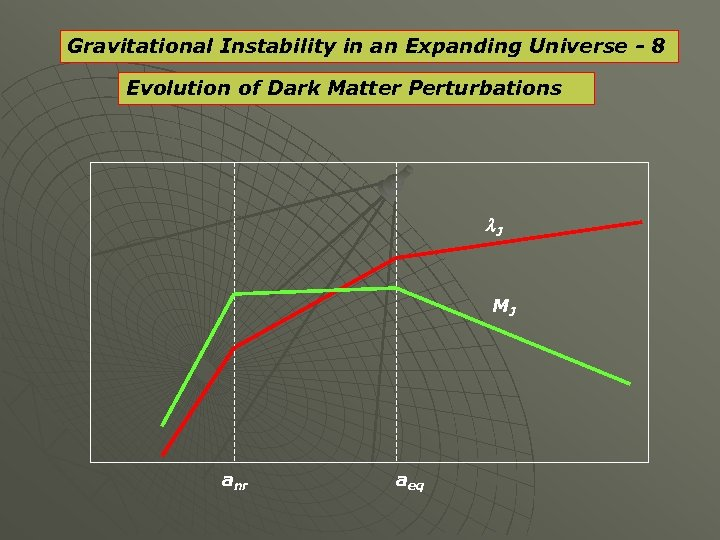Gravitational Instability in an Expanding Universe - 8 Evolution of Dark Matter Perturbations l. J MJ anr aeqGravitational Instability in an Expanding Universe - 9 Evolution of Baryonic Matter Perturbations Proceeding through analogous scaling relations With one major difference wrt DM: -Before the epoch of recombination, radiation and baryonic matter are tightly coupled, radiation providing the pressure of the coupled fluid. -After recombination, the pressure drops by many orders of magnitude to practically zero (that of a few 1000 K baryon fluid). - As a result, both the Jeans’ Length and Mass drop precipitously. Numerically: For: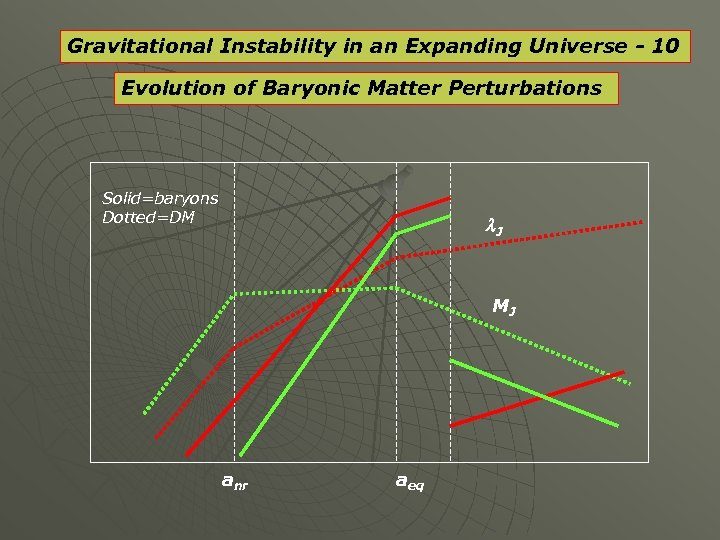Gravitational Instability in an Expanding Universe - 10 Evolution of Baryonic Matter Perturbations Solid=baryons Dotted=DM l. J MJ anr aeq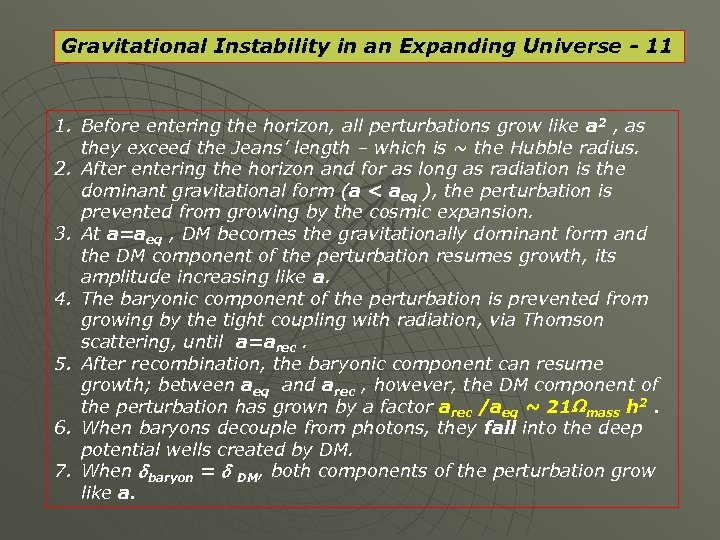Gravitational Instability in an Expanding Universe - 11 1. Before entering the horizon, all perturbations grow like a 2 , as they exceed the Jeans’ length – which is ~ the Hubble radius. 2. After entering the horizon and for as long as radiation is the dominant gravitational form (a < aeq ), the perturbation is prevented from growing by the cosmic expansion. 3. At a=aeq , DM becomes the gravitationally dominant form and the DM component of the perturbation resumes growth, its amplitude increasing like a. 4. The baryonic component of the perturbation is prevented from growing by the tight coupling with radiation, via Thomson scattering, until a=arec. 5. After recombination, the baryonic component can resume growth; between aeq and arec , however, the DM component of the perturbation has grown by a factor arec /aeq ~ 21 Wmass h 2. 6. When baryons decouple from photons, they fall into the deep potential wells created by DM. 7. When dbaryon = d DM, both components of the perturbation grow like a.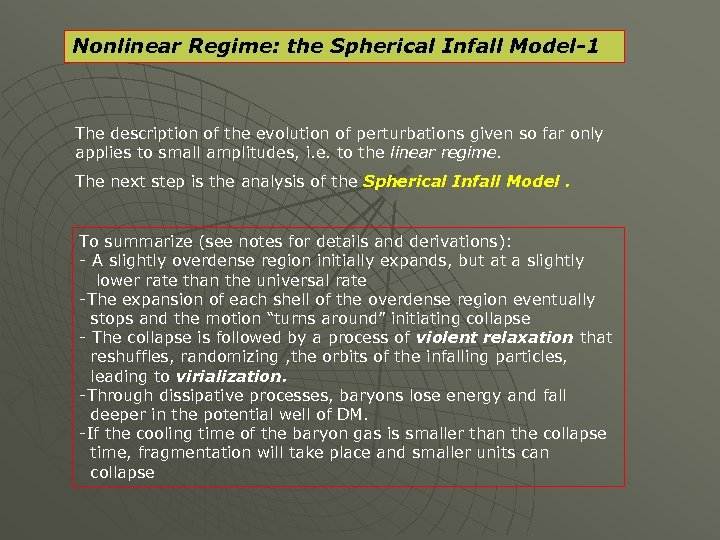Nonlinear Regime: the Spherical Infall Model-1 The description of the evolution of perturbations given so far only applies to small amplitudes, i. e. to the linear regime. The next step is the analysis of the Spherical Infall Model. To summarize (see notes for details and derivations): - A slightly overdense region initially expands, but at a slightly lower rate than the universal rate -The expansion of each shell of the overdense region eventually stops and the motion “turns around” initiating collapse - The collapse is followed by a process of violent relaxation that reshuffles, randomizing , the orbits of the infalling particles, leading to virialization. -Through dissipative processes, baryons lose energy and fall deeper in the potential well of DM. -If the cooling time of the baryon gas is smaller than the collapse time, fragmentation will take place and smaller units can collapse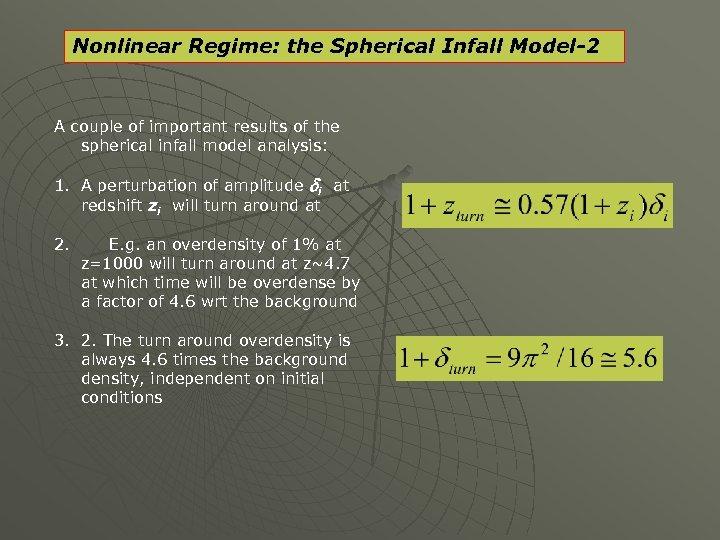Nonlinear Regime: the Spherical Infall Model-2 A couple of important results of the spherical infall model analysis: 1. A perturbation of amplitude di at redshift zi will turn around at 2. E. g. an overdensity of 1% at z=1000 will turn around at z~4. 7 at which time will be overdense by a factor of 4. 6 wrt the background 3. 2. The turn around overdensity is always 4. 6 times the background density, independent on initial conditions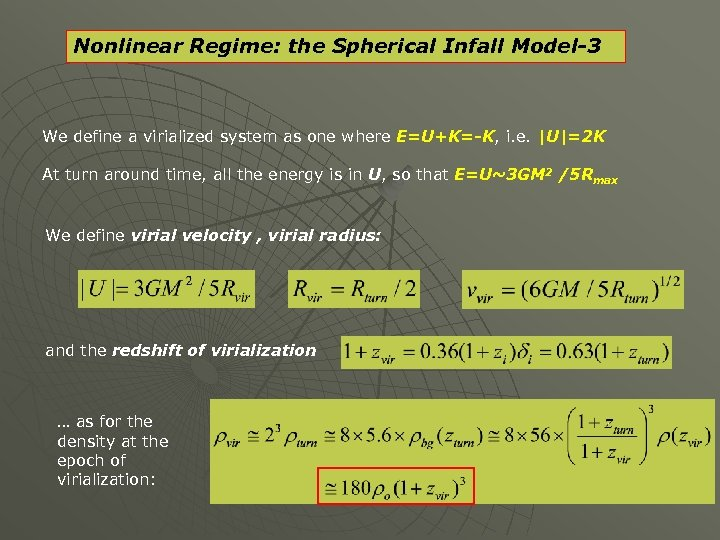Nonlinear Regime: the Spherical Infall Model-3 We define a virialized system as one where E=U+K=-K, i. e. |U|=2 K At turn around time, all the energy is in U, so that E=U~3 GM 2 /5 Rmax We define virial velocity , virial radius: and the redshift of virialization … as for the density at the epoch of virialization: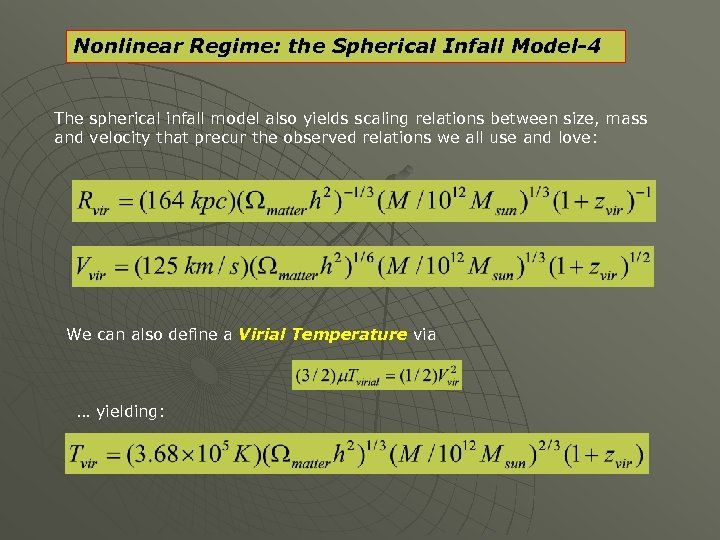Nonlinear Regime: the Spherical Infall Model-4 The spherical infall model also yields scaling relations between size, mass and velocity that precur the observed relations we all use and love: We can also define a Virial Temperature via … yielding: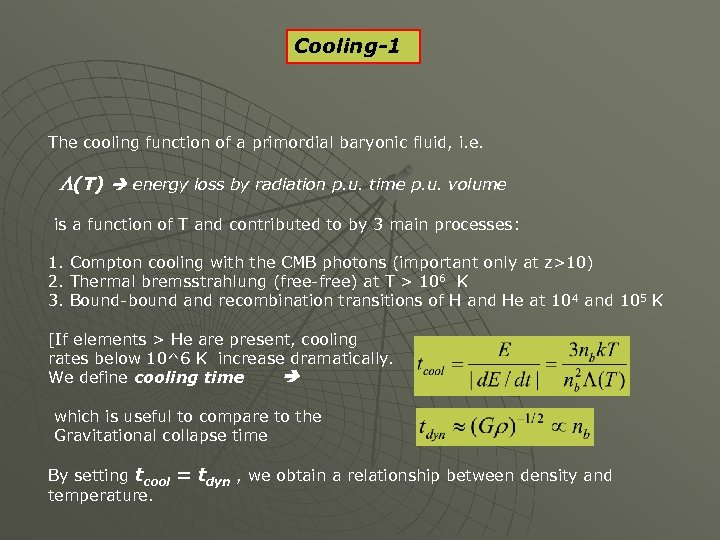Cooling-1 The cooling function of a primordial baryonic fluid, i. e. L(T) energy loss by radiation p. u. time p. u. volume is a function of T and contributed to by 3 main processes: 1. Compton cooling with the CMB photons (important only at z>10) 2. Thermal bremsstrahlung (free-free) at T > 106 K 3. Bound-bound and recombination transitions of H and He at 10 4 and 105 K [If elements > He are present, cooling rates below 10^6 K increase dramatically. We define cooling time which is useful to compare to the Gravitational collapse time By setting tcool = tdyn , we obtain a relationship between density and temperature.